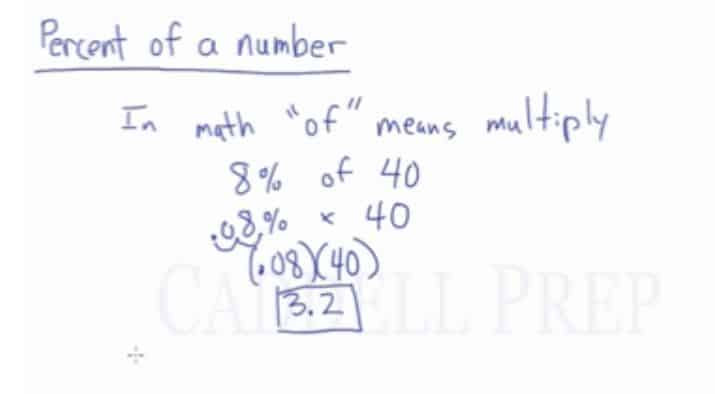In this video, we will be learning how to find a percent of a number using proportions. After you finish this lesson, view all of our Pre-Algebra and Algebra lessons and practice problems.

In math, “of” means multiply

Percent Formula:$\frac{Percent}{100}=\frac{Part (is)}{Whole(of)}$

## Example of Finding the Percent of a Number

What is 8% of 40?$\dfrac{8}{100}=\dfrac{x}{40}\leftarrow$ First we cross-multiply$\dfrac{320}{100}=\dfrac{100x}{100}\leftarrow$ Then we divide by 100 on both sides to isolate x$3.2=x$

## Example 1

What is$17\%$ of$30$?

First, we cross-multiply$\dfrac{17}{100}=\dfrac{x}{30}$

Then, we divide by$100$ on both sides to isolate$x$$\dfrac{510}{100}=\dfrac{100x}{100}$$5.1=x$

The answer is$5.1$

## Example 2

What percent of$50$ is$4.8$?

We have the “of” and the “is”. Our formula should look like this:$\dfrac{x}{100}=\dfrac{4.8}{50}$

Let’s cross multiply$x\times50$ is$50x$$4.8 \times 100$ is$480$

We have:$50x=480$

Now, let’s divide both sides by$50$$\dfrac{50x}{50}=\dfrac{480}{50}$$x=9.6$

Therefore, the answer is$9.6\%$

## Video-Lesson Transcript

In this video, we will be learning how to find a percent of a number using proportions.

In math, ‘of’ means ‘multiply‘.

So if you’re asked$8\%$ of$40$, it means$8\% \times 40$.

We can’t get the answer right away.

We have to change$8\%$ into a decimal.

So we have to move the decimal two spaces from the right to left.$8\%$ will become$0.08$ then we can multiply$0.08 \times 40 = 3.2$

Another way to find the percent of a number is the percent formula.

Percent formula is$\dfrac{Percent}{100} = \dfrac{Part (is)}{Whole (of)}$

What is$8\%$ of$40$?

Let’s use the formula$\dfrac{8}{100} = \dfrac{x}{40}$

Let’s cross-multiply$8 \times 40$ is$320$$x \times 100$ is$100x$

Now we have$320 = 100x$

To isolate$x$, let’s do the inverse operation.

We just have to divide both sides by$100$

And we get$3.2 = x$Which is the same answer using a different method.

To sum up, you can multiply or use the percent formula to get the answer if the missing value is the “is” or the part.

But if the missing value is the percent or the whole, you have to use the percent formula.

To give an example, let’s answer this question:

What percent of$40$ is$3.2$?

Here, we’re looking for the percent. We have the “of” and the “is”.

The formula is$\dfrac{Percent}{100} = \dfrac{Part (is)}{Whole (of)}$

Since we’re missing the percent, our formula should look like this$\dfrac{xt}{100} = \dfrac{3.2}{40}$

Then let’s cross multiply$x \times 40$ is$40x$$3.2 \times 100$ is$320$

Now, let’s do the inverse operation of multiplication which is division.

So, let’s divide both sides by$40$$\dfrac{40x}{40} = \dfrac{320}{40}$

The answer is$x = 8$

So the answer to what percent of$40$ is$3.2$?

The answer is$8\%$